Mobile QR Code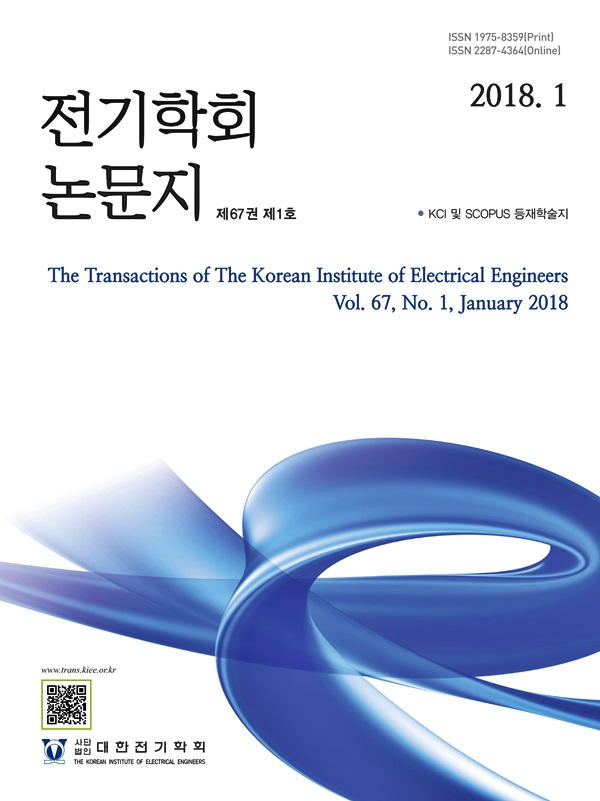The Transactions of the Korean Institute of Electrical Engineers

ISO Journal TitleTrans. Korean. Inst. Elect. Eng.

1. (Dept. of Electrical Engineering, Daejin University, Korea.)
2. (Dept. of Electrical Engineering, Daejin University, Korea.)

Forced Outage Rate, HVAC, HVDC, Availability, Power Conversion Station, Wind Turbine, Reliability

1. Introduction

Recently, it has focused on expanding the supply of renewable energy worldwide. In line with this, Korea is also preparing policies to actively respond through energy conversion, such as the 3020 implementation plan for renewable energy and 2050 carbon neutrality. Wind power generation is considered the most realistic alternative among renewable energy sources. In Korea, a wind power complex is being developed to expand the ratio of renewable energy generation, and the ratio of renewable energy generation is expected to increase to about 70% by 2050. Accordingly, more wind power complexes will be constructed. With the addition of wind power generators, it can act as a factor that degrades the reliability of the power system. The forced outage rate may vary depending on the configuration of the wind power generator and may affect the forced outage rate for each wind power generator arrangement. In addition, few studies have been conducted considering the forced outage rate of conversion stations from offshore wind power generators to power system connections.

When transmitting renewable energy, it can be divided into DC transmission and AC transmission, the DC power transmission method has advantages over the existing power transmission method, the AC method, due to various factors in the long- distance power transmission method. There are fewer losses and constraints compared to AC, the recently discussed lines including main land-Jeju transmission are all adopted. In the case of direct current transmission method, power transmission is performed by connecting the power conversion system to the existing AC transmissions are required. This is because power conversion facilities, which are facilities that convert from direct current transmission to alternating current transmission, have a high forced outage rate because they are composed DC power transmission facilities. However, there is not much reliability analysis in general involving the economic concept of determining system reliability.

In addition, the failure of the components of the facility cannot ignore the impact on the entire system. There is few research on the overall system forced outage rate considering the forced outage rate according to the serial and parallel structures of the system.

In this study, the system forced outage rate is calculated by dividing the forced outage rate for each element from the wind power generator to the power system connection by HVAC and VSC-HVDC transmission.

2. Calculation of the Forced Outage Rate

The system is connected in series and parallel to the power facility. In order to obtain the forced outage rate of the entire system, the forced outage rate for each component must be changed to availability, calculated, and then changed to the forced outage rate again. The following Equation (1) represents an equation for calculating the availability of a component by the forced outage rate of the component.

(1)
$A_{i}=1-FR(i)$

The failure of the entire system varies depending on the failure of parts of a specific facility and the structure constituting each facility. If any one of the components constituting the facility causes the failure of the entire system, it can be said that the system has serial parts. The reliability structure of the serial system can be expressed as shown in Figure 1 below (6).

그림. 1. 직렬 시스템의 신뢰도 구조

Fig. 1. Reliability structure of the serial system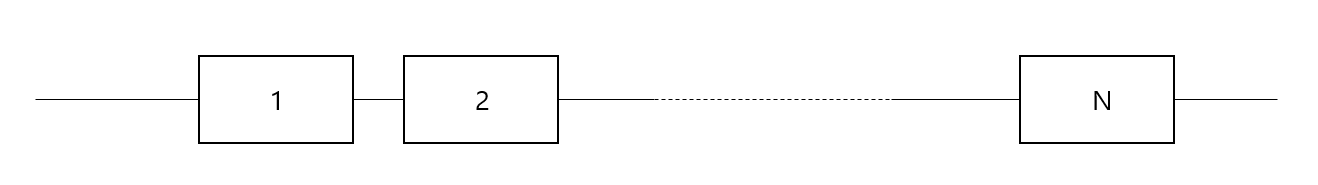Probabilistic, the reliability of the entire system is the probability of a serial system when a system consisting of multiple parts does not fail from part 1 to part. The reliability of the serial system is shown in Equation (2).

(2)
$A_{s}=A_{A}\bullet A_{B}\bullet\cdots\bullet A_{n}=\prod_{i=1}^{n}A_{i}$

The parallel system structure differs from the serial system structure in the calculation method. The reliability of the parallel system can be said that the system has parallel parts only when all the parts seeking facilities in the system fail. Therefore, in order not to fail the parallel system, at least one of the components in the system only needs to operate. The reliability structure of parallel systems can be expressed as shown in Figure 2 below (6).

그림. 2. 병렬 시스템의 신뢰도 구조

Fig. 2. Reliability structure of the parallel system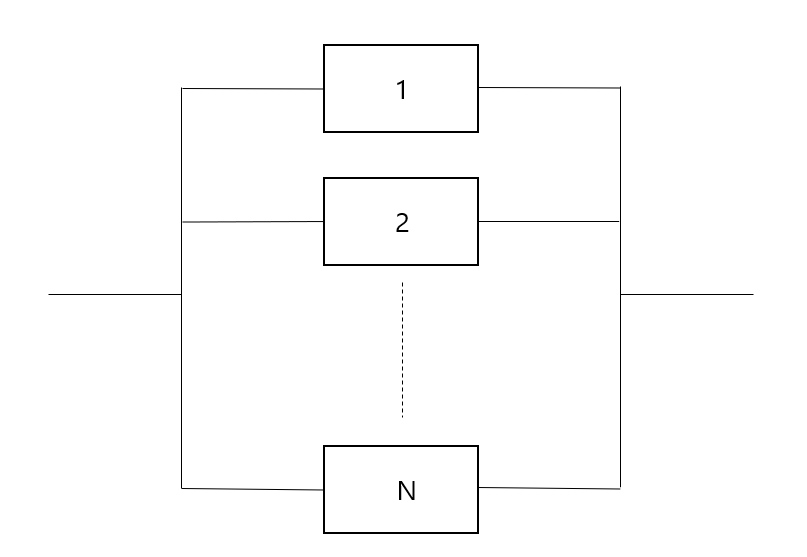The reliability of the parallel system is as shown in Eq. 3.

(3)
$A_{p}=1-\prod_{i=1}^{n}(1-A_{i})$

If the availability of the entire system is calculated using equations (2) and (3) and then converted into a failure rate again, it is possible to calculate the Forced Outage rate of the entire system.

Failure of each component of the system affects the forced outage rate of the entire facility. However, we need to understand the structure between components. If a failure of one component directly affects the failure of the whole system, it is a serial structure, and if all components are connected to the failure of the whole system, it has a parallel structure as an indirect failure. Therefore, Eq. 2 was applied for serial structures and Eq. 3 for parallel structures, and most of the systems were mixed as serial and parallel structures, so the forced outage rate was calculated using both Eq. 2 and (3).

3. Calculation of Forced Outage Rate of HVAC and VSC-HVDC Facilities

3.1 HVAC Model for calculating Forced Outage Rate

Figure 3 is a transmission system layout model for wind farms based on HVAC technology (5).

In Figure 3, A: Circuit breaker (33kV), B: Sea transformer (33kV/transmission voltage), C: Separator actuator, D: Circuit breaker (transmission voltage), E: Ground transformer (transmission voltage/400kV), F: Circuit breaker (400kV), and G:3 core XLPE submarine cable (transmission voltage). Table 1 shows each component and input data as forced outage rates.

표 1. HVAC 구성요소 별 고장률

Table 1. Forced Outage Rate of HVAC Components

 Component FOR[%] Transformer 33 [kV] 132 [kV] 200 [kV] 400 [kV] - 0.41 0.28 0.35 Breaker 33 [kV] 132 [kV] 220 [kV] 400 [kV] 0.53 0.20 0.20 0.17 Shunt Reactor 0.07 XLPE 0.5

3.2 VSC-HVDC Model for calculating Forced Outage Rate

HVDC is divided into current type and voltage type. Voltage- type HVDC have many advantages over current-type HVDC in the form of converting alternating current into direct current through switching using MMC(Modular Multilevel Converter) devices. Recent research on Voltage-type HVDC (VSC-HVDC) has been actively conducted.

그림. 4. VSC-HVDC 변환소 모델

Fig. 4. VSC-HVDC Power conversion station model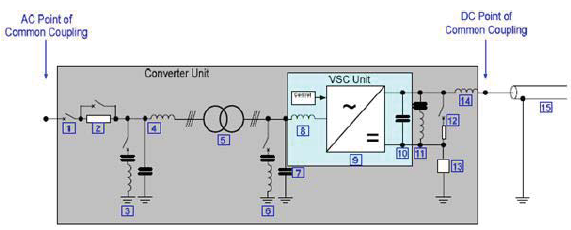Figure 4 shows 1. Circuit breaker, 2. Pre-insertion Resistors, 3. Transmission line-side harmonic filter, 4. Transmission line -side high-frequency filter, 5. Transformer, 6. Converter-side harmonic filter, 7. Converter-side high-frequency filter, 8. Topological Reactor, 9. VSC Unit, 10. VSC DC Capacitor, 11. DC Harmonic filter, 12. Dynamic braking system, 13. Neutral point ground, 14. DC reactor, 15. DC cable, or machined Transmission lines. The following Table 2 shows each component and input data as forced outage rates (7).

표 2. VSC-HVDC 구성요소 별 고장률

Table 2. Forced Outage Rate of VSC-HVDC Components

 Component FOR[%] Component FOR[%] Breaker 0.866 Transformer 0.191 AC, DC Filter 0.699 Dynamic braking system 0.001 DC Trans- mission Line 0.950 Reactor 0.312 Capacitor 0.002

For reactors, capacitors and filters, it is assumed that they have a common forced outage rate, and for transmission line forced outage rate data, they appear in units of forced outage rate per DC conductor rather than km.

3.3 Forced Outage Rate Calculation of HVAC and VSC-HVDC

As a result of calculating the system-wide forced outage rate of HVAC and VSC-HVDC using equations (2) and (3), the forced outage rate of HVAC systems can be 1.44[%] at voltage level 132[kV], 1.18[%] at 220[kV], and 1.19[%] at 400[kV]. Calculating the forced outage rate of VSC-HVDC power conversion station results in a forced outage rate of 3.57[%]. Here, the forced outage rate of components not specified in Table 2 is assumed to be zero. The forced outage rate of the HVDC Transmission line was based on the forced outage rate data of one DC conductor. As a result of the calculation, the overall forced outage rate of the assumed voltage-type HVDC system is calculated as 7.02[%] as shown in Figure 5. Comparing the forced outage rates of HVAC and VSC-HVDC, VSC-HVDC was found to be 7.02[%], higher than HVAC.

그림. 5. VSC-HVDC 구성

Fig. 5. Configuration of VSC-HVDC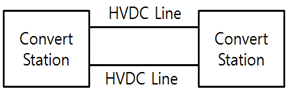4. The components and failure rate of wind power generators

Figure 6 shows the 5 MW wind power generator currently used for wind power generation (1).

그림. 6. 5[MW] REpower MM92 풍력발전시스템

Fig. 6. 5[MW] REpower MM92 wind turbine system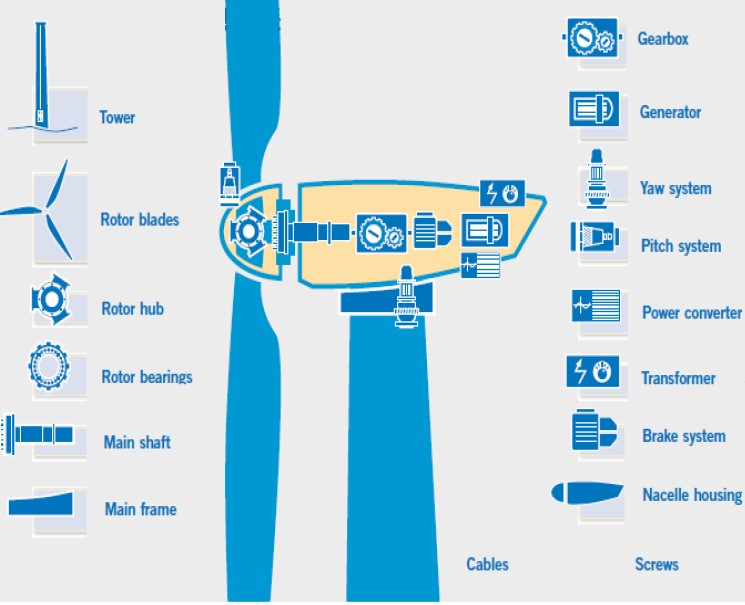Figure 6 shows wind power generation facilities, Tower, Rotor blades, Rotor hub, Rotor bearings, Main frame, and Gearbox, Generator, Yaw system, Peach system, Power converter, Transformer, and Brake system from the left.

The following Table 3 shows each component and failure rate (1). The failure rate of the internal cable of the wind power generator was 0.008 [No./year]. It was calculated by changing the failure rate in Table 3 to the availability rate.

표 3. 풍력발전기 구성요소 및 고장률[횟수/년]

Table 3. Components of wind power generators and failure rates [No./year]

 Component FR Component FR Tower 0.144 Gearbox 0.179 Rotor blades 0.174 Generator 0.15 Rotor hub 0.138 Yaw system 0.013 Rotor bearings 0.01 Pitch system 0.013 Main shaft 0.043 Power converter 0.068 Main frame 0.011 Transformer 0.14 Nacelle housing 0.012 Brake system 0.013

그림. 7. 풍력발전기의 직렬 및 병렬 배치

Fig. 7. Serial and Parallel configuration of wind power generators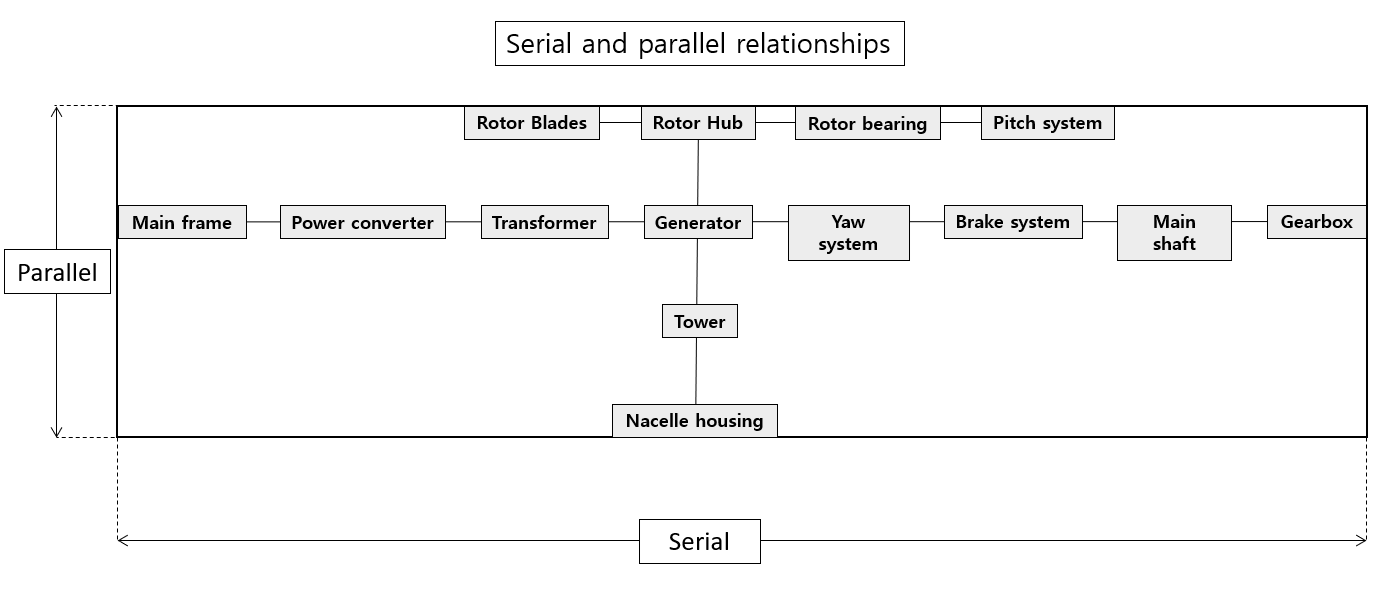As shown in Figure 7, the serial and parallel configuration of the wind power generator components was confirmed, and as a result of calculating the failure rate of each wind power generator component using equations (2) and (3), the failure rate of one wind power generator can be calculated as 3.1%.

5. Case study on the calculation of wind power generation system failure rate

5.1 Model for the failure rate of wind power plants

Figure 8 is the plan diagram for the construction of Jeju Hallim Offshore Wind Power Complex.

그림. 8. 제주 한림해상풍력발전단지 조성계획도

Fig. 8. Jeju Hallim Offshore Wind Power Complex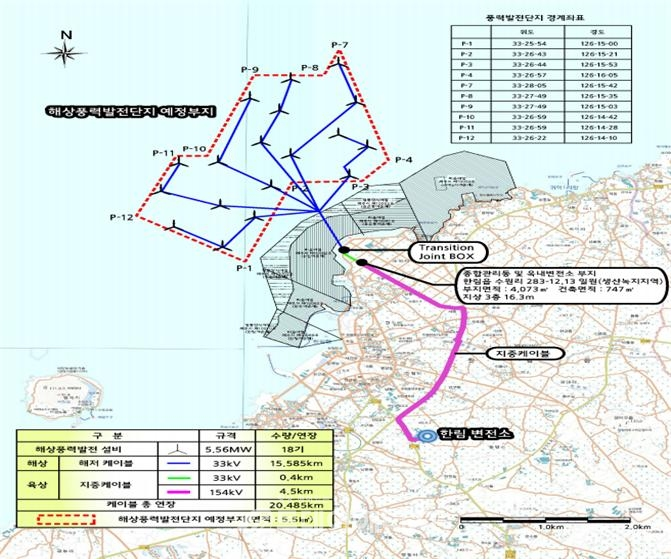As shown in Fig. 9, the wind power generators of the offshore wind power complex are partially connected in series, but each generator acts as a parallel connection element when calculating the failure rate.

그림. 9. 풍력발전기 구성도

Fig. 9. Composition of wind power generators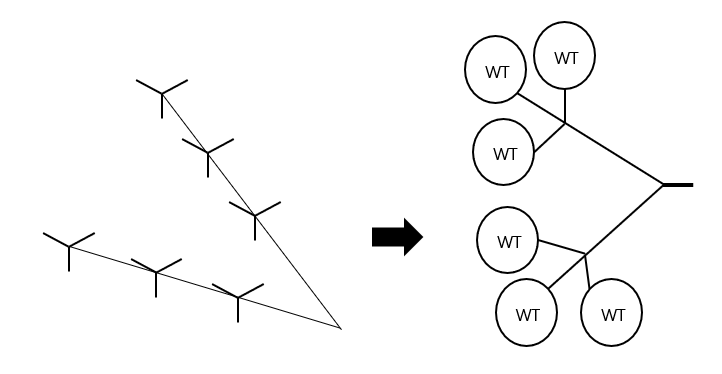5.2 Calculating the failure rate of wind power generation systems by number

Figure 10 shows the wind power generator to the power system connection point. The cables connecting the wind power generator and the convert station connect the inside and the outside. The failure rates of internal and external cables used at this time are 0.0074 [No./yearㆍkm], 0.0089 [No./yearㆍkm] (4). The failure rate of the Convert Station in Figure 8 was calculated as 3.57[%] as a result of calculating through equations (2) and (3).

그림. 10. 풍력발전기 수에 따른 VSC-HVDC 시스템 구성(1~4대)

Fig. 10. System Configuration by the number of wind power generators (1 to 4 units)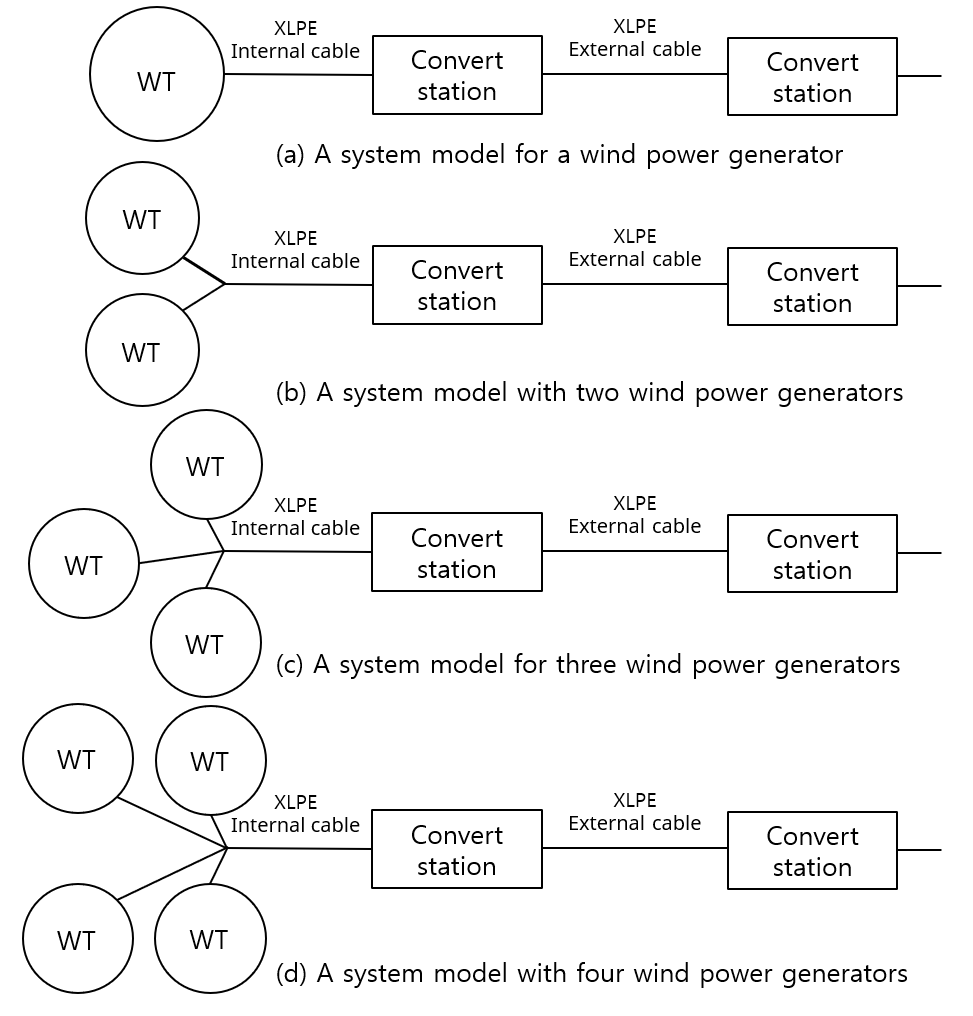Figure 11 shows the results of calculating the failure rate by the number of wind power generators during HVAC and VSC-HVDC transmission. The failure rate decreased as the wind power generator increased. For one unit, about 40% and about 17% for two or more units, and the failure rate was high for VSC-HVDC transmission.

그림. 11. 풍력발전기 수에 따른 HVAC 와 VSC-HVDC 시스템 고장률

Fig. 11. HVAC & VSC-HVDC system failure rate by number of wind power generators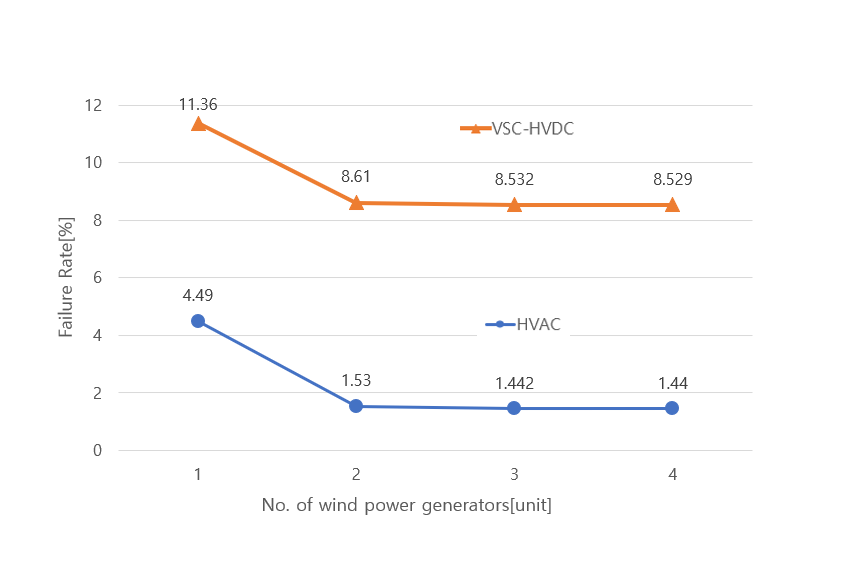When the system is configured as shown in Fig. 10, since the power generation source structure is applied as a parallel structure, output power does not occur only when all generators fail. As the number of wind power generators increases, the overall failure rate in the system decreases. Therefore, as the generator increases, the reliability of the entire system increases.

6. Conclusion

This paper calculated the system failure rate according to the number of wind power generators using the failure rate by component for the serial and parallel structures of the system.

1. The power transmission method was divided into HVAC transmission and VSC-HVDC transmission, and as a result of calculating the failure rate for each component by checking the serial and parallel structure of each power transmission method, it was confirmed that the failure rate was high because VSC-HVDC included a convert station.

2. As a result of checking the structure of the wind power generator and calculating the failure rate of the generator through the failure rate of each component, it was confirmed to be 3.1[%].

3. According to each power transmission method, a model system was configured from wind power generator to power connection point, and the failure rate of 1 to 4 generators was calculated, and it was verified that the failure rate decreased as generators were added.

However, due to the lack of related data, overseas data and overseas models were used, and if Korean data were used, the failure rate suitable for Korean systems can be calculated.

In this paper, the failure rate of power transmission systems, including wind power generators, was calculated. The components of each facility were checked and the failure rate of the entire system was calculated through the failure rate of each component. However, it was difficult to obtain domestic data for the model and failure rate of each facility, so foreign data from the reference was used. In addition, the application of the variable called external factors is insufficient. If actual field data are applied in the future, more accurate results will be obtained.

In the future, various case studies will be supplemented to calculate the more accurate failure rate of the system, including large-scale offshore wind power generation complexes, based on the data analyzed in this study.

References

1
Fateme Dinmohammadi, Mahmood Shafiee, 2013, A fuzzy- FMEA risk assessment approach for offshore wind turbines, International Journal of Prognostics and Health Management 4.13, pp. 59-682
Bertz Tourgoutian, Alexander Yanushkevich, Riaan Marshall, 2015, Reliability and availability model of offshore and onshore VSC-HVDC transmission systems3
R. en Allan, R. Billinton, 1996, Reliability Evaluation of Power Systems, New York: Plenum Press4
Jong-Nam Won, 2013, A Study on Siting of HVAC Offshore Substation for Wind Power Plant using Submarine Cable Cost Model, Journal of the Electrical Society, Vol. 62, No. 4, pp. 451-4565
LAZARIDIS, 2005, Economic Comparison of HVAC and HVDCSolutions for Large Offshore Wind Farms underSpecial Consideration of Reliability6
Kim Won-kyung, 2005, Theory and Practice of Reliability Engineering, Friendship Center7
Tourgoutian. Bezrt, al et, 2015. 7, Reliability and availability model of offshore and onshore VSC-HVDC transmission system, 11th IET International Conference on AC and DC Power Transmission8
Zadkhast Sajjad, 2010. 7, Reliability Evaluation of an HVDC Transmission System Tapped by a VSC station, IEEE Transctions on Power Delivery, Vol. 25, No. 39
Electrotechnical Commission International, 2014, IEC 62747: Terminology for voltage-sourced converters (VSC) for high- voltage direct current (HVDC) sytems, IEC10
Gyu-Hyoung Kim, Hui-Won Jeong, Junmin CHA, 2021, Forced Outage Rate Calculation of HVAC and VSC-HVDC, Proceedings of APAP2021저자소개

He received B.S. degree in electrical engineering from Daejin University in 2020.

He is a master's student.

His research interests are reliability of power system, renewable energy.

E-mail : 4rbgud@naver.com

He received B.S., M.S. degree in electrical engineering from Daejin University in 2008 and 2020.

He is a Ph.D. Candidate at the Daejin University in 2020.

Since 2021, he has been worked as a manager of NEXPO.

His research interests are reliability of power system, load forecasting, interconnection power line and arc protection & elimination system.

E-mail : jwh6993@naver.com

He received B.S., M.S. and Ph.D. degree in power system engineering from Korea University in 1989, 1991 and 1996.

Since 1996, He has been on the faculty of Daejin University, Pocheon, Korea, where he is a professor.

His research interests are reliability of power system including ESS & reneable energy, maintenance scheduling of generators and load forecasting,

E-mail : chamin@daejin.ac.kr